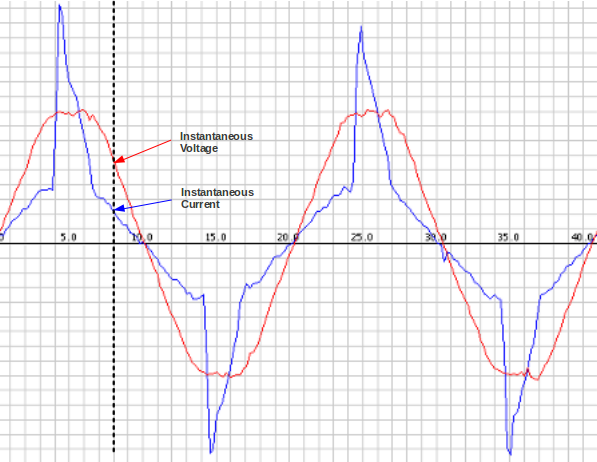# AC Power Theory - Arduino Maths

## Instantaneous Voltage and Current

As the name suggests, AC Voltage and current continually alternate. If we draw a picture of the voltage and current waveform over time, it will look something like the image below. Depending on the type of load consuming power, the current waveform - blue in the diagram below - is what you get if you look at a typical laptop computer power supply. (There’s an incandescent light bulb present, as well).

The image was made by sampling the mains voltage and current at high frequency, rather faster than we can do in the emonTx or Arduino. Using emonLib, we can make approximately 100 measurements every 20 milliseconds if sampling only current, or approximately 50 if sampling both voltage and current. We’re limited by the Arduino analog read command and calculation speed.

Each individual sample is an instantaneous voltage or current reading.## Calculating Real Power on an Arduino

Real power is the average of instantaneous power. The calculation is relatively straightforward.

First we calculate the instantaneous power by multiplying the instantaneous voltage measurement by the instantaneous current measurement. We sum the instantaneous power measurement over a given number of samples and divide by that number of samples:

```for (n=0; n<number_of_samples; n++)
{
// inst_voltage and inst_current calculation from raw ADC input goes here

inst_power = inst_voltage * inst_current;

sum_inst_power += inst_power;
}

real_power = sum_inst_power / number_of_samples;

```

## Root-Mean-Square (RMS) – Voltage

The root-mean-square (r.m.s) value is important because it represents the value of a direct voltage or direct current that will give the same heating effect in a resistor.

The root-mean-square is calculated in the way the name suggests. First we square the quantity, then we calculate the mean and finally, the square-root of the mean of the squares, this is how it is done:

```for (n=0; n<number_of_samples; n++)
{
// inst_voltage calculation from raw ADC input goes here.

squared_voltage = inst_voltage * inst_voltage;

sum_squared_voltage += squared_voltage;
}

mean_square_voltage = sum_squared_voltage / number_of_samples;
root_mean_square_voltage = sqrt(mean_square_voltage);
```

## Root-Mean-Square (RMS) – Current

Same as the RMS voltage calculation:

```for (n=0; n<number_of_samples; n++)
{
// inst_current calculation from raw ADC input goes here.

squared_current = inst_current * inst_current;

sum_squared_current += squared_current;
}

mean_square_current = sum_squared_current / number_of_samples;
root_mean_square_current = sqrt(mean_square_current);

```

## Apparent Power

`apparent_power = root_mean_square_voltage * root_mean_square_current;`

As RMS voltage is generally a fixed value such as: 230V (+10% -6% in the UK), it’s possible to approximate apparent power without making a voltage measurement by setting the RMS voltage to the nominal supply voltage, 120 V in N. America, 230V in Europe or 240 V in the UK. 110 V and 220 V are also common values but check and use your local voltage. This is a common practice used by commercially available energy monitors.

## Power Factor

The definition of power factor is:

```power_factor = real_power / apparent_power;
```

That’s the fundamentals of AC power measurement on the emonTx or an Arduino.

## EmonLib

We have packaged these calculations into an Arduino library called EmonLib to simplify Arduino energy monitor sketches. There are two versions. The methods in the original version perform the calculations over a given number of samples or mains cycles and return the average values. This is the simplest version to study for anyone new to discrete maths and energy monitoring. The “Continuous Monitoring” version, as the name suggests, operates continuously in the background and reports the average values at intervals. The libraries can be found on GitHub here: https://github.com/openenergymonitor/EmonLib and https://github.com/openenergymonitor/EmonLibCM.